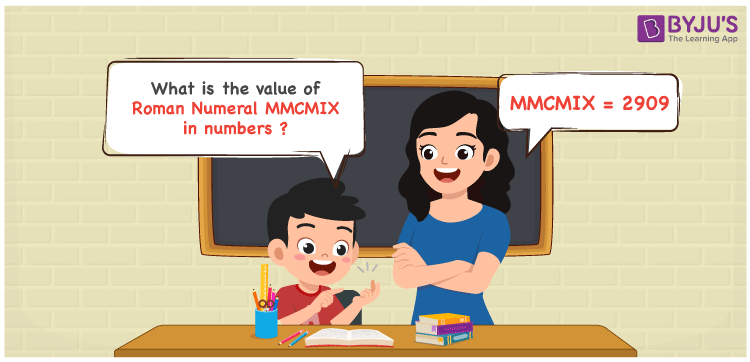Checkout JEE MAINS 2022 Question Paper Analysis : Checkout JEE MAINS 2022 Question Paper Analysis :

# MMCMIX Roman Numerals

MMCMIX Roman Numerals is 2909. The Roman numerals MMCMIX is expanded and indicated in numbers here. Students will be able to curate perfect answers for questions on the Roman numerals if they read this article thoroughly. Therefore, the roman numerals MMCMIX is written as 2909 in numeric form.

 Number Roman Numeral 2909 MMCMIX## How to Write MMCMIX Roman Numerals in Numbers?

Learn the process followed to write MMCMIX in numbers using the steps below.

MMCMIX = M + M + (M – C) + (X – I)

MMCMIX = 1000 + 1000 + (1000 – 100) + (10 – 1)

MMCMIX = 2909

## Video Lesson on Roman Numerals## Frequently Asked Questions on MMCMIX Roman Numerals

### Why should 2909 be written in Roman Numerals as MMCMIX?

We know that

MMCMIX = M + M + (M – C) + (X – I)

MMCMIX = 1000 + 1000 + (1000 – 100) + (10 – 1)

MMCMIX = 2909

### What is 3000 – 91?

We know that

3000 – 91 = 2909

Hence, 3000 – 91 is 2909 which is written as MMCMIX.

### What is the remainder when MMCMIX is divided by XVIII?

We know that

MMCMIX = 2909

XVIII = 18

The remainder when MMCMIX is divided by XVIII is XI.